Function Repository Resource:

# LeastSquaresPlot

Plot data together with a visualization of the squared errors compared to a fit

Contributed by: Jon McLoone
 ResourceFunction["LeastSquaresPlot"][data] plots data together with the linear best fit and the squared errors. ResourceFunction["LeastSquaresPlot"][data,n] uses the order-n best fit. ResourceFunction["LeastSquaresPlot"][data,expr,var] also plots the curve given by expr and the variable var.

## Details and Options

ResourceFunction["LeastSquaresPlot"] has the same options as ListPlot, with the following addition:
 "SquareStyle" {Opacity[0.2]} graphics directives to specify the style of the error squares

## Examples

### Basic Examples (3)

Show the squared errors of a linear fit to some data. A tooltip on each square gives its area:

 In:=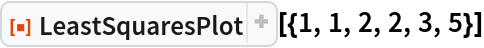Out=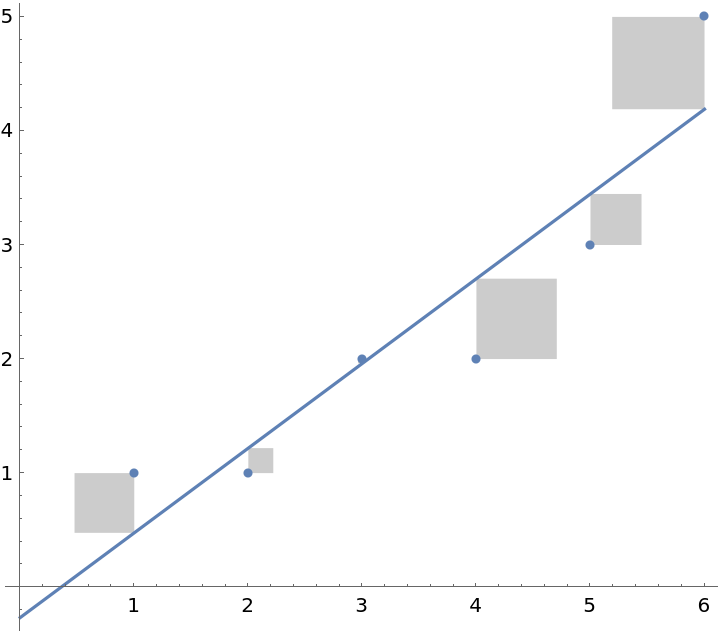Show the squared errors of a quadratic fit to some data. A tooltip on each square gives its area:

 In:=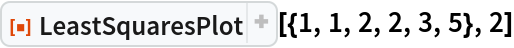Out=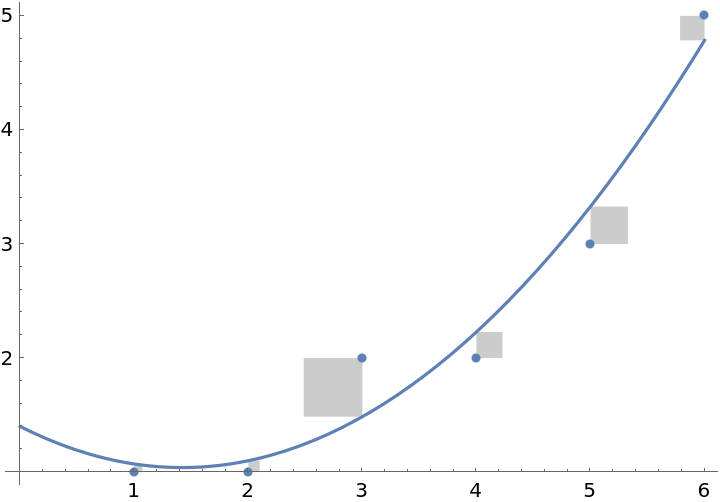Compare the errors to the parabola y=x2/10:

 In:=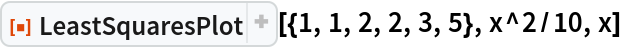Out=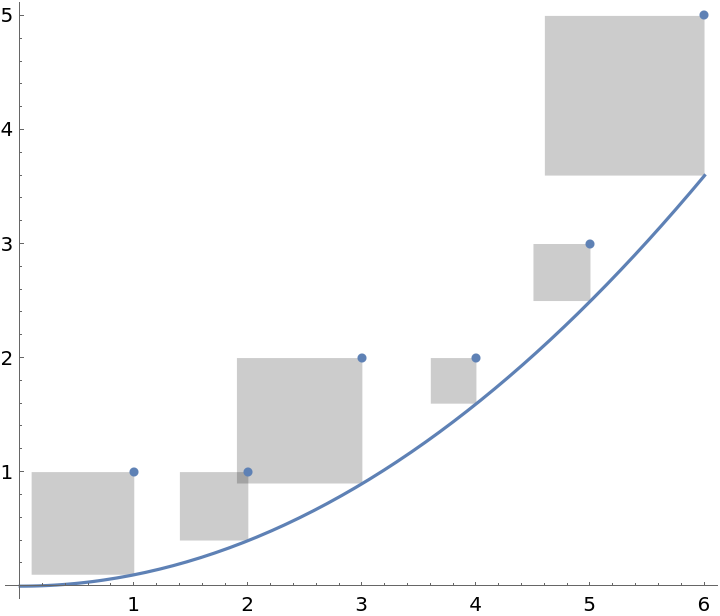### Options (1)

#### SquareStyle (1)

Change the style of the error squares:

 In:=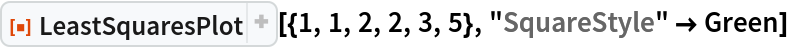Out=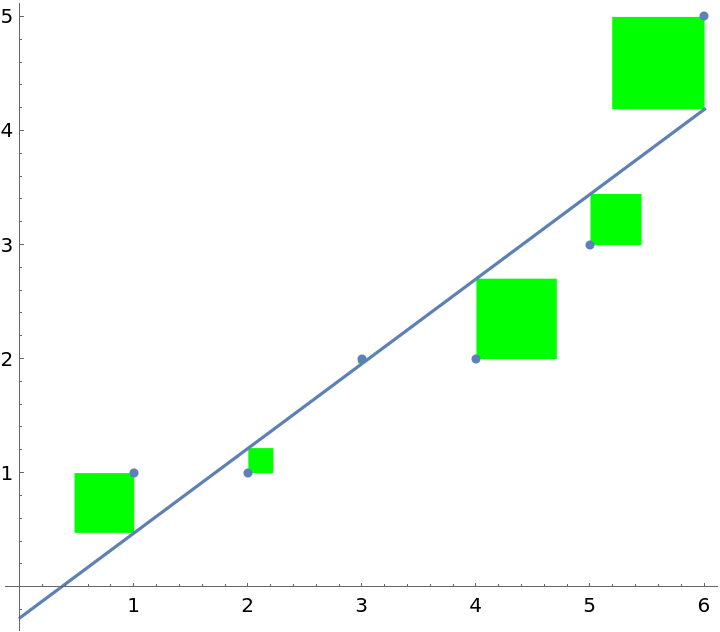Jon McLoone

## Version History

• 1.0.1 – 13 February 2023
• 1.0.0 – 27 March 2020